# Quiz 10: Developing Project Cash Flows

Capital investment is one of the crucial decisions in a business. Fixed assets are capital asset. Investment in fixed asset is considered here. Initially lump sum money is employed to buy the asset. Then asset renders service during its lifetime. Revenue is earned and cash flows are generated. Firm has to decide whether investment is justified or not. Different techniques are available for taking such decisions. They are known as capital budgeting technique. Most of the techniques are using cash flow figures for taking decisions. Thus calculation of correct cash flow is very crucial. Different techniques are available for taking such decisions. They are known as capital budgeting techniques. Most of the techniques are using cash flow figures for taking decisions. Thus calculation of correct cash flow is very crucial. The problem requires initial investment of \$65,000 to buy a machine. It has 6 years life. Resale value of machine after 6 years is zero. So, entire \$65,000 will be depreciated during 6 years. Depreciation is the value lost for using machine in production. In tax rule, modified accelerated cost recovery system (MACRS) is used for each group. Further the method has assumed that capital asset will provide maximum service in initial years. So, high rate of depreciation is recommended. It is 200% of normal rate for asset life below 15 years. This rate is 150% for life of 15 years or more. The problem is related with purchase of machine. Cost price is \$65,000. Salvage value recoverable from machine after six years life is zero. So, entire \$65,000 is depreciable. Machine is depreciable for 5 years life span. So, in each year equal percentage will be recovered. At the start of year zero, depreciable value of asset is 100% of initial cost. Divide it by 5 years. It is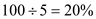. Since life is less than 15 years, take 2005 of normal rate. It is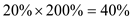. So in first year, depreciation rate is 40%. However MACRS assumes that machine is introduced at the middle of year 1. So 50% first year depreciation rate will be applicable. It is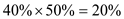. Therefore, full 100% will be recovered in 6 years. After charging 20% depreciation in year 1, leftover book value of machine is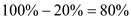. For 2 nd year, depreciation rate applicable will be 40% of 80% book value. It is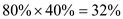In this manner, calculate MACRS for remaining years. Remember MACRS rate cannot be less than straight line rate. It is a rate which is ascertained by dividing book value using number of year's life left. So, calculation of MACRS is shown in the table below: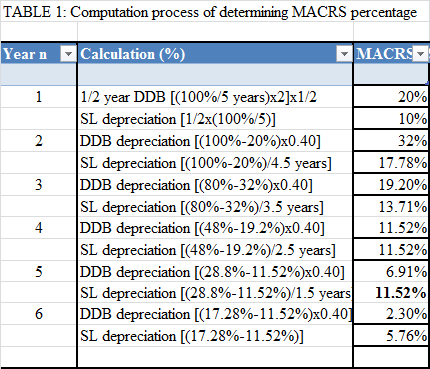Now consider cash flow from machine. Actual life of the machine is 6 years. In each year, it will save labor cost of \$35,000. Also annual operating and maintenance cost is \$12,000. So net cash inflow is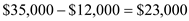. It is revenue before tax. From the above figure, deduct depreciation. You will get taxable net income. Now deduct tax to get net income after tax. Finally add back depreciation. It is needed to ascertain cash flow. Note that depreciation is a book entry. No cash is paid. So after add back, you will get annual cash flow.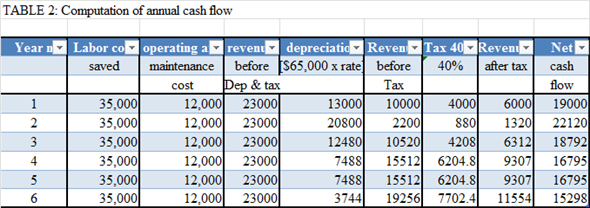Finally apply net present worth concept to judge acceptability of project. Procedures are- 1. Consider annual cash flow. 2. Calculate annual discounting factor of each year. It is present value of one dollar receivable in future. Formula is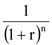. Here 'r' is discounting rate. It is minimum rate of return required to be earned for survival. Future cash flow period is 'n'. 3. Multiply annual net cash flow by the corresponding discounting factor. It will give present value of yearly net cash flow. 4. Add all annual present value calculated in step 3 to get gross present worth. 5. Finally deduct initial investment to get net present worth. If net present worth is positive, then project is accepted. Here discounting rate is 15%. So net present worth calculation is made and following table is prepared.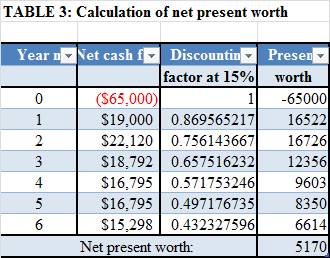Since net present worth is positive, project is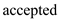Machine should be purchased.
Options of Buy or borrow and lease: The manager has to decide whether to borrow funds for the fixed asset or to purchase it from market or it can be taken on lease. While taking this decision, the important aspects which are required to be considered are the total value and the life span, in case of purchase. On the other hand, the rental payments and the production level at the end of the period are considered in case of lease. Net Cash Flows: The net cash flows are defined as the difference between the cash inflows and outflows of a company at a particular period of time. In the present case, the company, CC had the fixed overhead cost of \$7.5 million with the MACRS 7 year class. 1. It is needed to determine the treat the R D expenditures. It can be seen under two situations: Situation 1: When the firm decides not to follow the project, then the expense in 2015 would be calculated as done below:This amount will be written off and considered as the tax savings. Situation 2: When the firm decides to follow the project, it would incur the opportunity cost of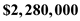. 2. It is required to determine the depreciation schedules of the assets. The land would have no depreciation. Building would fall under the depreciation class of 39- MACRS property and Equipment would fall under the depreciation class of 7- MACRS property. 3. It is required to determine the taxable gains for each assets. For the land asset , the taxable gain would be calculated as done below:For the building asset , the taxable gain would be calculated as done below:For the Equipment asset , the taxable gain would be calculated as done below:Working notes for the calculation of Depreciation on building and equipment are given below: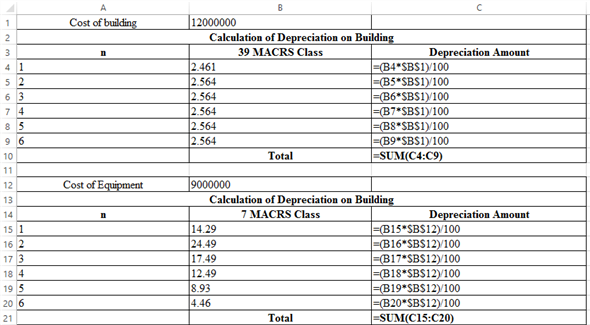The corresponding values are: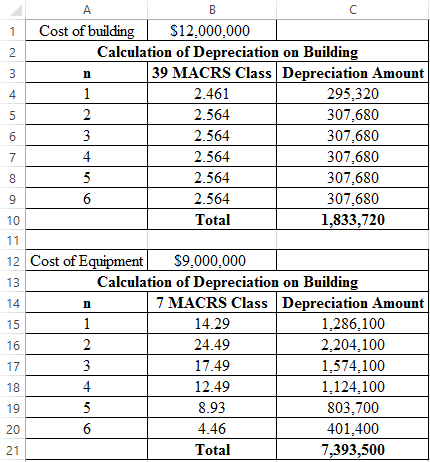4. It is required to determine the amount of working capital requirement in each period. For the year 2017, it can be calculated with the formula given below:For the year 2018, it can be calculated with the formula given below: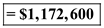5. It is needed to find the project cash flow by determining the Net present worth criteria and IRR in the excel file. It can be calculated with the appropriate formula in the spreadsheet given below: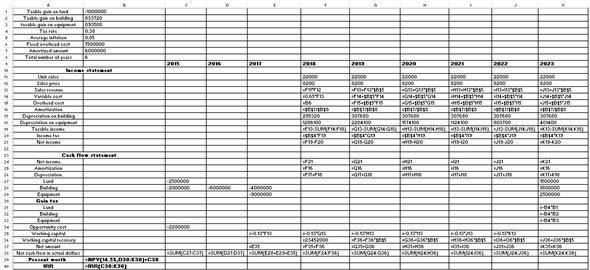The corresponding values are: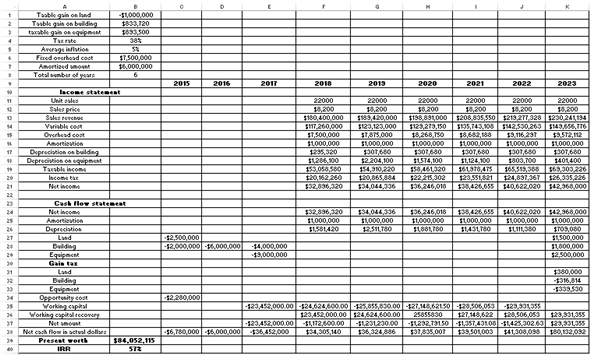Hence, the calculated present worth is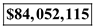.
It is given that the initial cost of the robot is \$210,000 and it will help in saving \$150,000 to the firm. The robot will be depreciated under MACRS as a five-year property. The useful life of the robot is 7 years and the salvage value of the robot is \$60,000. The marginal tax rate is 35% per year and the MARR is 15%. BTCF is the before tax cash flow. It shows the amount that the firm earns before paying any taxes. Depreciation is the amount of loss in value the tool due to wear and tear. MACRS depreciation is calculated on the original value of machine. The table below shows the rate of depreciation under MACRS: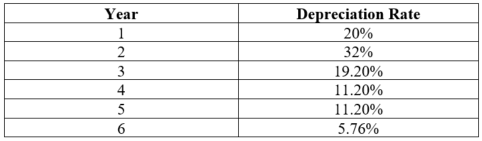Taxable income is calculated by deducting the amount of depreciation from the before tax cash flow.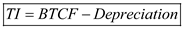Tax is the amount of tax that must be paid. The combined income tax rate is 39%.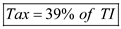After-tax cash flow is the cash flow of the firm after deducting the taxes from the before-tax cash flow.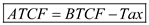Following is the screenshot of the formulae used in calculation of depreciation, taxable income and after-tax cash flow: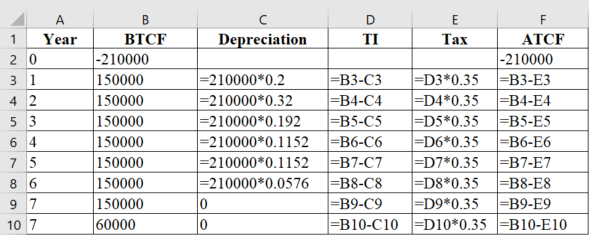The result image is as follows: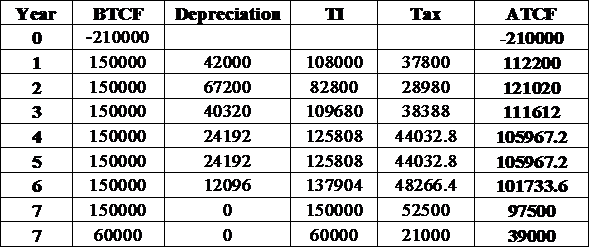The last column in the table is the salvage value of the robot. Following is the formula to calculate the after-tax salvage value:Thus, the after-tax salvage value of the robot is \$39,000.